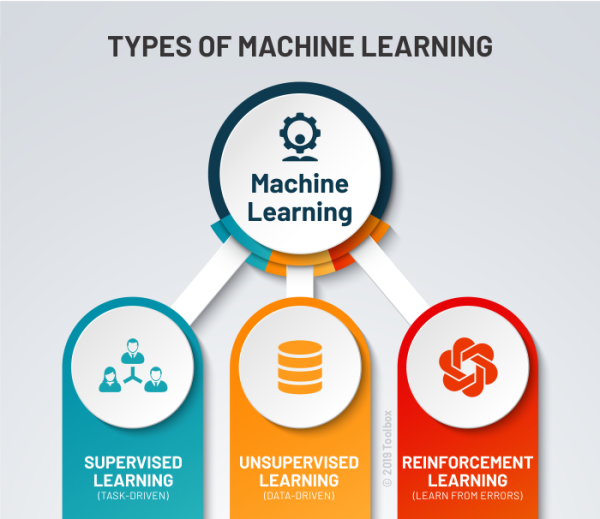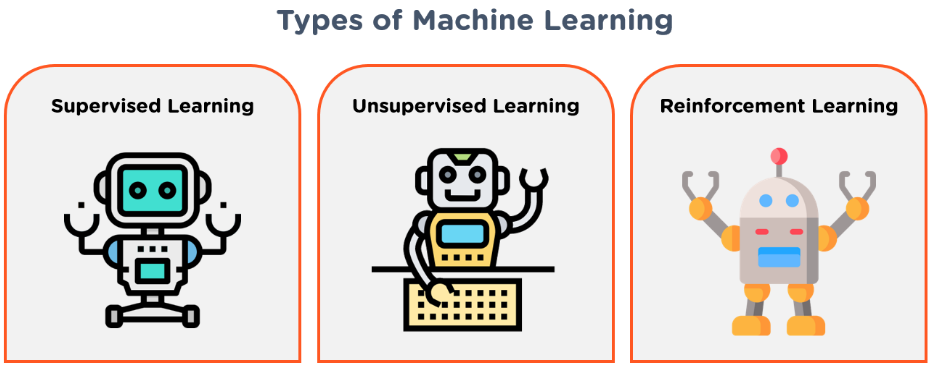# Types of Machine Learning## 1. Supervised Learning:In this type of algorithm, there is a target variable that we wish to predict. This target variable is dependent on various independent variables. A function is generated using this set of variables that gives the desired outputs. The model is trained until the training dataset achieves the desired accuracy.

This category can further be divided into Classification, Regression, and Forecasting.

Supervised learning is the most common type of machine learning, where the algorithm learns from labeled data. In this approach, the training dataset consists of input data (features) and their corresponding output labels. The goal is to learn a mapping function that can predict the output label for new, unseen inputs accurately. Supervised learning can be further classified into two main categories:

### Classification:

In this type of supervised learning, the dependent variable is of 2 or more types.

Algorithms that come under classification:

For example:

Filter an email into “spam” or “not spam” by looking at existing observational data.

Netflix’s recommendation system also uses classification algorithms to recommend which movie a person should watch next.

Examples of classifications algorithms:

1. KNN
2. Decision Trees
3. Random forest

And many more.

Classification is the task of predicting discrete class labels. The algorithm learns from labeled examples and assigns new instances to predefined classes. For example, an email spam filter is a classification problem, where emails are classified as “spam” or “not spam” based on their features.

### Regression:

In this type of supervised learning, the machine learning model understands the relationship among various variables and helps estimate the value of a  new dependent variable when certain independent variables are available.

For example:

Predicting the price of a house, it is common knowledge that the larger the house, the more expensive it will be. To predict its price, we can give the regression model a dataset of areas of various houses and their prices then, when a new house’s area is sent in as input, the model can predict its price.

Training Dataset:

500 yards cost \$100k

700 yards cost \$140k

2000 yards cost \$400k

After the training input area of 1000 yards is given, the model will successfully tell that its cost will be \$200k.

This example used linear regression where the area and cost graph plot will create a line on the 2-dimensional plane. More details in further articles.

Examples of regression algorithms:

1. Linear Regression
2. Polynomial Regression
3. Ridge Regression
4. Lasso Regression

And many more.

Regression involves predicting continuous numerical values. The algorithm learns the relationships between input variables and their corresponding output values. For instance, predicting house prices based on features like area, location, and number of rooms is a regression problem.

### Forecasting:

In this type of supervised learning, the model studies the historical data and helps create a prediction.

For example:

Various multi-million dollar companies like Goldman Sachs and Morgan Stanley use forecasting algorithms to predict stock prices.

This can also be used to predict anything from the weather to the currently unstable crypto market just the accuracies vary.

Examples of forecasting algorithms:

1. LSTM
2. ARIMA
3. XGBoost
4. Prophet

And many more.

Common algorithms used in supervised learning include decision trees, random forests, support vector machines (SVM), logistic regression, and neural networks. Supervised learning finds applications in various fields, such as image recognition, speech recognition, sentiment analysis, and medical diagnosis.

## 2. Unsupervised LearningHere there isn’t a target variable to predict or estimate. This method is used to sort the dataset into various groups or segments. No human is there to provide instructions on how to divide the dataset. Instead, the algorithm determines what correlations exist by analyzing the data. This is especially beneficial when there are large datasets to organize.

Unsupervised learning deals with unlabeled data, where the algorithm explores the data’s inherent structure or patterns without predefined output labels. Unlike supervised learning, unsupervised learning aims to discover meaningful information or clusters within the data. Unsupervised learning can be categorized into two main types:

As more data is assessed, the ability to make decisions improves.

This is of 2 main subtypes:

### Clustering:

Similar data is segmented into several groups based on different criteria of this type. Clustering is responsible for dividing the dataset into server groups and then performing analysis on each cluster to find patterns.

Clustering is used in Medical imaging, Social Network analysis, etc.

This method can be used to divide music into genres. Music with heavy drum sounds and guitar solos would be divided into Rock, while songs with saxophone or trumpet sounds would go into the Jazz segment.

Examples of Clustering algorithms:

1. KMeans
2. Spectral Clustering
3. Affinity Propagation

And more.

Clustering algorithms group similar data points together based on their inherent similarities or distances. It helps in identifying natural groupings within the data. An example of clustering is customer segmentation, where customers with similar characteristics or purchasing behaviors are grouped together.

### Dimension Reduction:

This type of unsupervised learning is used to reduce the number of variables being considered when working with large datasets.

For example:

The DNA in humans contains various types of protein and gene segments, this number is over 10,000, and it would take a large number of computational resources to process such a dataset.

In such a case, we use dimensional reduction to create a method where the information provided by the most relevant parts is considered for computation.

Examples of Dimension Reduction Algorithms:

1. PCA
2. t-SNE
3. Singular Value Decomposition

And more.

Dimensionality reduction techniques aim to reduce the number of input features while preserving important information. It helps in simplifying complex datasets and visualizing data in lower-dimensional spaces. Principal Component Analysis (PCA) and t-distributed Stochastic Neighbor Embedding (t-SNE) are common dimensionality reduction techniques.

Unsupervised learning has applications in various domains, including customer behavior analysis, anomaly detection, recommendation systems, and image segmentation.

## 3. Reinforcement Learning:The main focus in reinforcement learning is on the learning process, where the algorithm is provided with instructions, parameters, and end values. Here the algorithm follows the roles, explores different possibilities, and then evaluates to find the most optimal.

This is a type of trial and error method to achieve the best possible result.

The easiest example to understand is the case of a bot playing a game of chess. Chess follows certain rules and has the desired outcome ( to create a checkmate condition). Every match the bot plays it learns many more possibilities and understands the game better.

There are 2 types of reinforcements given to the algorithm:

1. Positive: This is when a good event occurs, and the algorithm is prompted to increase the frequency of such events and have positive behavior. This helps maximize performance.
2. Negative: This is when a negative condition occurs and is to be stopped or avoided. This creates a minimum behavioral standard for the model.

Reinforcement Learning can be used for Industrial Automation, and self-driving cars also work on this principle. They learn to avoid accidents (Negative reinforcement) and are encouraged to go on the path that takes the least amount of time (Positive reinforcement).

Reinforcement learning involves an agent learning to make decisions through interactions with an environment. The agent learns to take actions to maximize a reward signal by trial and error. It receives feedback in the form of rewards or penalties based on its actions, enabling it to learn optimal strategies over time. Reinforcement learning is composed of three main components:

a. Agent: The entity that learns and interacts with the environment.

b. Environment: The external world or problem space in which the agent operates.

c. Rewards: Feedback or signals that the agent receives from the environment to evaluate its actions.

Reinforcement learning has found significant applications in robotics, game playing, autonomous vehicles, and recommendation systems.

## Conclusion

Supervised learning, unsupervised learning, and reinforcement learning are the major types of machine learning approaches. Supervised learning deals with labeled data and focuses on predicting class labels or numerical values. Unsupervised learning works with unlabeled data and aims to discover patterns or groupings within the data. Reinforcement learning involves an agent learning to make decisions through interactions with an environment to maximize rewards. Understanding these different types of machine learning provides a foundation for selecting appropriate algorithms and techniques for various real-world applications.